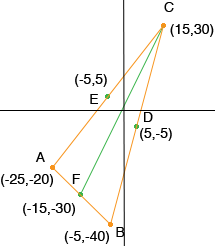Andrea secondary (grade 10) student I am trying to find the centriod of a triangle. I have been given the three vertices of the triangle: A (-25, -20), B (-5, -40), and C (15, 30). I believe I calculated the correct midpoints: AB(E) (-5, 5), BC(D) (5, -5), and AC(F) (-15, -30). When I graphed these points I came up with the solution (-5, -10), but I can't seem to write the answer out (equations of the lines, etc) correctly. Any help would be greatly appreciated! Yours truly, Andrea. Andrea, My diagram shows that E is the midpoint of CA, F is the midpoint of AB and D is the midpoint of BC.I will write the equation of the line joining C and F, using the point slope form. The line is (y - y1) = m(x - x1) (y - 30) = m (x - 15) and m = (30 - (-30))/(15 - (-15)) = 60/30  = 2 Thus the equation of the line is (y - 30) = 2 (x - 15) or y = 2 x Penny